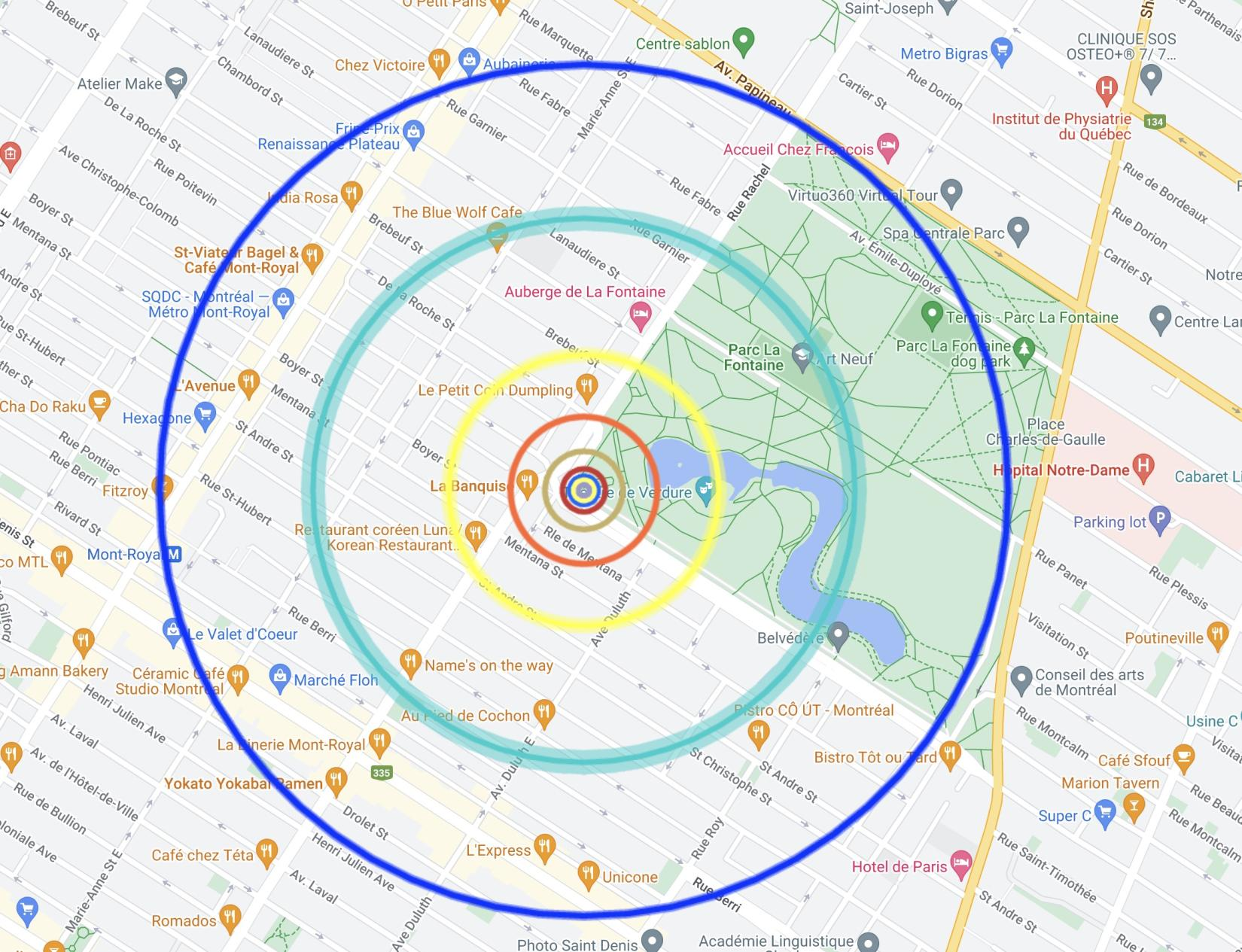# Build an Outdoor Scale Model Solar System

An outdoor demonstration of the distance and size of the planets.

In this outdoor activity, students will learn to build a scale model of the Solar System. They'll learn how big the Sun is compared to the planets and how far apart everything is in space.

## Basics

• Duration: 1 hour
• Ages: 8+
• Price: \$250
• Location: in-person in Montréal, large park needed

## Québec Curriculum Connections

### Mathematics

#### Geometry

A. Space

• 1. Gets his/her bearings and locates objects in space (spatial relationships)
• 2. Locates objects in a plane
• 3. Locates objects on an axis (based on the types of numbers studied)

#### Measurement

A. Lengths

• 1. Compares lengths
• 2. Constructs rulers
• 4. Estimates and measures the dimensions of an object using conventional units
• a. metre, decimetre and centimetre

G. Time

• 1. Estimates and measures time using conventional units
• 2. Establishes relationships between units of measure

### Science and Technology

#### Earth and Space

D. Systems and interaction

• 2. System involving the sun, the Earth and the moon
• Associates the sun with the idea of a star, the Earth with the idea of a planet and the moon with the idea a natural satellite
• 4. Seasons
• a. Describes the changes to the environment throughout the seasons (temperature, amount of daylight, type of precipitation)
• Explains the sensations experienced (hot, cold, comfortable) with regard to temperature measurements

### Mathematics

#### Arithmetic

B. Fractions (using objects or drawings)

• 3. Matches a fraction to part of a whole (congruent or equivalent parts) or part of a group of objects, and vice versa
• 4. Identifies the different meanings of fractions (sharing, division, ratio)
• 5. Distinguishes a numerator from a denominator
• 6. Reads and writes a fraction
• 7. Compares a fraction to 0, 1⁄2 or 1
• 8. Verifies whether two fractions are equivalent

### Science and Technology

#### Material World

E. Techniques and instrumentation

• 1. Use of simple measuring instruments
• Appropriately uses simple measuring instruments (rulers, dropper, graduated cylinder, balance, thermometer, chronometer)

#### Earth and Space

B. Energy

• 1. Sources of energy
• a. Explains that the sun is the main source of energy on Earth

E. Techniques and instrumentation

• 2. Use of simple measuring instruments
• a. Appropriately uses simple measuring instruments (e.g. rulers, dropper, graduated cylinder, balance, thermometer, wind vane, barometer, anemometer, hygrometer)

### Mathematics

#### Arithmetic

B. Fractions (using objects or drawings)

• 4. Identifies the different meanings of fractions (sharing, division, ratio)
• 7. Compares a fraction to 0, 1⁄2 or 1
• 8. Verifies whether two fractions are equivalent
• 9. Matches a decimal or percentage to a fraction
• 10. Orders fractions with the same denominator
• 11. Orders fractions where one denominator is a multiple of the other(s)

C. Fractions

• 1. Uses objects, diagrams or equations to represent a situation and conversely, describes a situation represented by objects, diagrams or equations (use of different meanings of addition, subtraction and multiplication by a natural number)

### Science and Technology

#### Material World

C. Forces and motion

• 3. Gravitational attraction on an object
• a. Describes the effect of gravitational attraction on an object (e.g. freefall)

E. Techniques and instrumentation

• 1. Use of simple measuring instruments
• Appropriately uses simple measuring instruments (rulers, dropper, graduated cylinder, balance, thermometer, chronometer)

#### Earth and Space

B. Energy

• 2. Transmission of energy
• a. Describes methods for transmitting thermal energy (e.g. radiation, convection, conduction)

D. Systems and interaction

• 3. Solar system
• a. Recognizes the main components of the solar system (sun, planets, natural satellites)
• b. Describes the characteristics of the main components of the solar system (e.g. composition, size, orbit, temperature)

### Science and Technology

#### The Earth and Space

C. Astronomical phenomena

• 1. Concepts related to astronomy
• a. Universal Gravitation
• i. Defines gravitation as a force of mutual attraction between bodies
• 2. Solar system
• a. Characteristics of the solar system
• i. Compares some of the characteristics of the planets in our solar system (e.g. distances, relative size, composition)

### Science and Technology

#### The Earth and Space

C. Astronomical phenomena

• 3. Space
• a. Scale of the universe
• i. Astronomical unit
• Defines an astronomical unit as the unit of length corresponding to the average distance between the Earth and the Sun
• ii. Light year
• Defines light year as a unit of length corresponding to the distance travelled by light in one Earth year
• iii. Location of the Earth in the universe
• Compares the relative distance between different celestial bodies (e.g. stars, nebulae, galaxies)
• b. Conditions conducive to the development of life
• i. Describes conditions conducive to the development or maintenance of life (e.g. presence of a gaseous atmosphere, water, energy source)

## What Your Class Will Learn

• how big the Sun is compared to the planets
• how far apart the planets are
• how to measure using a tape measure, and a digital map
• basics of scale models and how they can represent large things

## Activity

To start, we'll measure how big the park or field that is available to us using Google Maps. From there, we'll use a Solar System Model Calculator to determine how far away the Sun and Neptune can be.Once calculated, we'll have the sizes and distances of the planets. Using plasticine, we'll build each planet (however small), and then head outside.

Augmented reality will be used to show the size of the Sun. Then, we'll measure out how far each planet is.

## Book Now

Fill out the form below and let's get in touch! You'll receive an email confirmation, then I'll follow-up to confirm a date and anything special you'd like for your presentation.

Contact Form